# Section 6. Pointers and Linked Lists

Section materials curated by Kylie Jue and Nick Bowman, drawing upon materials from previous quarters.

This week’s section exercises delve deep into the details of pointers and memory management in C++. This is an opportunity to get down and dirty in the nitty gritty of things and to get closer towards gaining ultimate power over your computer! You will also gain valuable practice with linked lists, which are a new way of storing and organizing data that takes advantage of the power of pointers. Linked lists are definitely a tricky subject, but if you draw lots of diagrams and really nail down your pointer fundamentals, you'll be on the road to success. The topics covered in section this week will show up on the next assignment.

Remember that every week we will also be releasing a Qt Creator project containing starter code and testing infrastructure for that week's section problems. When a problem name is followed by the name of a `.cpp` file, that means you can practice writing the code for that problem in the named file of the Qt Creator project. Here is the zip of the section starter code:

For all the problems in this handout, assume the following structures have been declared:

``````struct Node {
int data;
Node *next;
};

struct StringNode {
string data;
StringNode *next;
};

struct DoubleNode {
double data;
DoubleNode *next;
};
``````

You can also assume the following utility functions have been defined as well:

``````/* Prints the contents of a linked list, in order. */
void printList(Node* list) {
for (Node* cur = list; cur != nullptr; cur = cur->next) {
cout << cur->data << endl;
}
}

/* Frees all the memory used by a linked list. */
void deleteList(Node* list) {
while (list != nullptr) {
/* Store where to go next, since we're about to blow up our linked
* list node.
*/
Node *next = list->next;
delete list;
list = next;
}
}
``````

## 1) A Series of Unfortunate References

Topics: Pointers and references

What is the output of the following code snippet?

``````void VFD(int *duncan, int isadora, int& quigley) {
(*duncan) = 1;
}

int main() {
int sunny = -6;
int klaus = 21;
int violet = 2;
VFD(&sunny, violet, klaus);
cout << sunny << " " << klaus << " " << violet << " " << endl;
return 0;
}
``````

1 -84 2

Note that the `violet` variable will not change since it is passed by value into the `VFD` function. However, both the `sunny` and `klaus` variables are affected by the changes in the `VFD` function. The `klaus` (`quigley`) variable is passed by reference so changes will persist in the original function. The address of the `sunny` variable is passed into `VFD` to the pointer variable `duncan`, and since the value at `duncan` is being edited via derefencing `(*duncan)`, the value of `sunny` also changes.

## 2) Some Pointers on Cats

Topics: Pointer tracing and memory diagrams

Trace through the following function and draw the program’s memory at the designated spot. Indicate which variables are on the stack and which are on the heap, and indicate orphaned memory. Indicate with a question mark (?) memory that we don’t know the values of.

``````struct Lion {
int roar;
int *meow;
int purr;
};

struct Savanna {
int giraffe;
Lion cat;
Lion *kitten;
};

Lion *explore(Savanna *prairie) {
prairie = new Savanna;
prairie->cat.roar = 6;
prairie->kitten->roar = 8;
prairie->kitten->meow = &(prairie->kitten->purr);
}

void kittens() {
Savanna *habitat = new Savanna;
habitat.giraffe = 3;
habitat.kitten = nullptr;
habitat = habitat;
habitat.kitten = explore(habitat);
habitat.kitten->roar = 4;

// DRAW THE MEMORY AS IT LOOKS HERE
}
``````

Orphaned memory (memory on the heap that we no longer have access to but that was not freed) is represented with dotted lines. For a full walkthrough of the solution, check out: https://tinyurl.com/CatsPointers.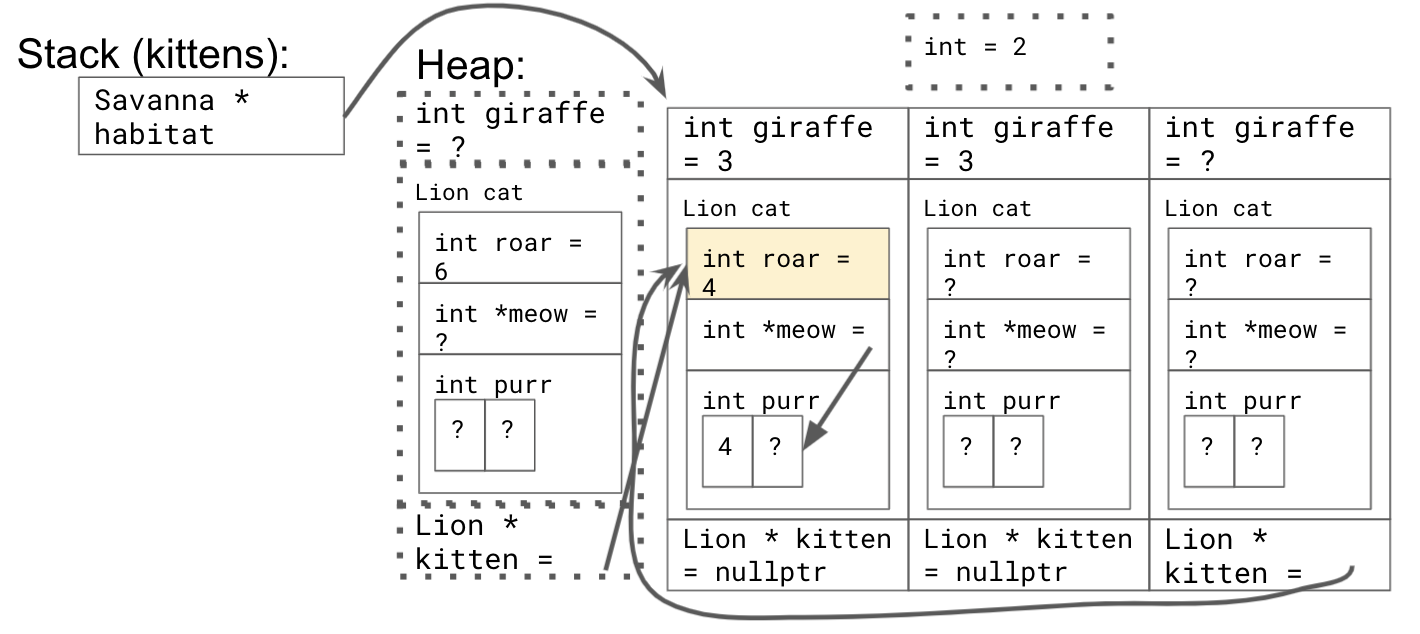## 3) What's the Code Do?

For each of the following diagrams, draw a picture of what the given nodes would look like after the given line of code executes. Does any memory get orphaned as a result of the operations? Assume the `Node` struct is the same as struct covered in lecture that stores integers.

Diagram 1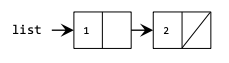Code Snippet 1

``````list->next = new Node;
list->next->data = 3;
``````

Diagram 2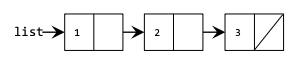Code Snippet 2

``````list->next->next = nullptr;
``````

Example 1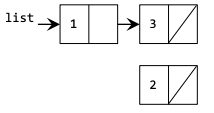This code produces one orphaned node with data 2.

Example 2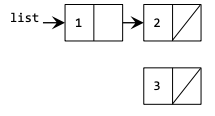This code produces one orphaned node with data 3.

For each of the following diagrams, write the code that will produce the given "after" result from the given "before" starting point by modifying the links between the nodes shown and/or creating new nodes as needed. There may be more than one way to write the code, but do not change the data field of any existing node. If a variable doesn't appear in the "after" picture, it doesn't matter what value it has after changes are made.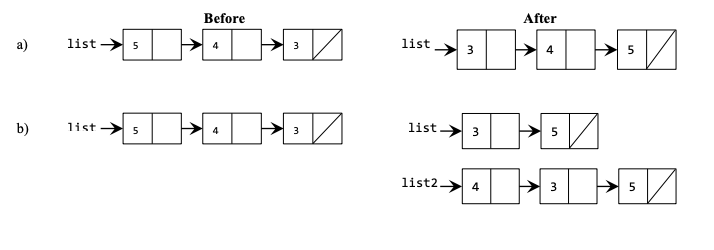a)

``````Node *temp = list->next->next;
temp->next = list->next;
list->next->next = list;
list->next->next->next = nullptr;
list = temp;
``````

b)

``````list->next->next->next = list;
list = list->next->next;
Node *list2 = list->next->next;
list->next->next = nullptr;
``````

## 5) Linked List Mechanics (`warmup.cpp`)

This section handout is almost exclusively about linked lists, so before we jump into some of their applications, let’s start off by reviewing some of the basic mechanics about how they work!

To begin with, let’s imagine we have a linked list of integers. Write a function

`int sumOfElementsIn(Node* list);`

that adds up the values of all the elements in the linked list. Write this function two ways – first, do it iteratively; then, do it recursively. Which one did you think was easier to write? Why?

Next, write a function

`Node* lastElementOf(Node* list);`

that returns a pointer to the last element of a linked list (and reports an error if the list is empty). Again, write this function two ways, iteratively and recursively. Which one did you think was easier to write?

Summing List Elements

``````/* Iterative version */
int sumOfElementsIn(Node* list) {
int result = 0;
for (Node* curr = list; curr != nullptr; curr = curr->next) {
result += curr->data;
}
return result;
}

/* Recursive version. */
int sumOfElementsIn(Node* list) {
/* The sum of the elements in an empty list is zero. */
if (list == nullptr) return 0;

/* The sum of the elements in a nonempty list is the sum of the elements in
* the first node plus the sum of the remaining elements.
*/
return list->data + sumOfElementsIn(list->next);
}
``````

Finding the Last List Element

``````/* Iterative version */
Node* lastElementOf(Node* list) {
if (list == nullptr) error("Empty lists have no last element.");

/* Loop forward until the current node next pointer is null. That’s the
* point where the list ends.
*/

Node* result = list;
while (result->next != nullptr) {
result = result->next;
}
return result;
}

/* Recursive version. */
Node* lastElementOf(Node* list) {
/* Base Case 1: The empty list has no last element. */
if (list == nullptr) error("Nothing can come from nothing.");

/* Base Case 2: The only element of a one-element list is the last element. */

if (list->next == nullptr) return list;

/* Recursive Case: There’s at least two nodes in this list. The last element
* of the overall list is the last element of the list you get when you drop
* off the first element.
*/
return lastElementOf(list->next);
}
``````

## 6) All out of Sorts (`sorted.cpp`)

Write a function that takes in a pointer to the front of a linked list of integers and returns whether or not the list that's pointed to is in sorted (nondecreasing) order. An empty list is considered to be sorted. You should implement your code to match the following prototype

``````bool isSorted(Node* front)
``````
``````bool isSorted(Node* front) {
if (front != nullptr) {
Node* current = front;
while (current->next != nullptr) {
if (current->data > current->next->data) {
return false;
}
current = current->next;
}
}
return true;
}
``````

Content for the following problems will be covered in lecture on Friday, August 6.

## 7) Recursive Merge (`merge.cpp`)

For Assignment 3, you wrote an iterative version of the merge operation to merge two sorted queues into one big sorted queue. Now, we're going to revisit the merge operation but with a couple of tweaks to the algorithm and to the data structures in play.

Your task is to write a recursive function `merge` that accepts two sorted linked lists and returns a pointer to a merged, sorted list. For example, suppose your function is given the following lists:

`{ 1, 4, 5, 10, 11 } { 2, 4, 6, 7, 8 }`

A call to your function should return a pointer to the following list:

`{ 1, 2, 4, 4, 5, 6, 7, 8, 10, 11 }`

Constraints: Do not swap data values or create any new nodes to solve this problem; you must create the merged list by rearranging the links of the lists passed to your function. Do not use auxiliary structures like arrays, vectors, stacks, queues, etc., to solve this problem. You should implement your code to match the following prototype

``````Node* merge(Node* a, Node* b)
``````
``````Node* merge(Node* a, Node* b) {
if (a == nullptr) {
return b;
}
if (b == nullptr) {
return a;
}

if (a->data <= b->data) {
a->next = merge(a->next, b);
return a;
} else {
b->next = merge(a, b->next);
return b;
}
}
``````

## 8) Tracing Pointers by Reference

One of the trickier nuances of linked lists comes up when we start passing around pointers as parameters by reference. To better understand exactly what that’s all about, trace through the following code and show what it prints out. Also, identify any memory leaks that occur in the program.

``````void confuse(Node* list) {
list->data = 137;
}

void befuddle(Node* list) {
list = new Node;
list->data = 42;
list->next = nullptr;
}

void confound(Node* list) {
list->next = new Node;
list->next->data = 2718;
list->next->next = nullptr;
}

void bamboozle(Node*& list) {
list->data = 42;
}

void mystify(Node*& list) {
list = new Node;
list->data = 161;
list->next = nullptr;
}

int main() {
Node* list = /* some logic to make the list 1 -> 3 -> 5 -> null */

confuse(list);
printList(list); // defined at beginning of section handout

befuddle(list);
printList(list);

confound(list);
printList(list);

bamboozle(list);
printList(list);

mystify(list);
printList(list);

freeList(list); // defined at beginning of section handout
return 0;
}
``````

Let’s go through this one step at a time.

• The call to `confuse` updates the first element of the list to store 137, so the call to `printList` will print out 137, 3, 5. Although the argument is passed by value, because both pointers point to the same Node in memory, the original list will also see a different value.
• The call to `befuddle` takes its argument by value. That means it’s working with a copy of the pointer to the first element of the list, so when we set list to be a new node, it doesn’t change where the list variable back in main is pointing. The node created in this function is leaked, and the next call to `printList` will print out 137, 3, 5.
• The call to `confound` takes its argument by value. However, when it writes to list->next, it’s following the pointer to the first element of the linked list and changing the actual linked list node it finds there. This means that the list is modified by dropping off the 3 and the 5 (that memory gets leaked) and replacing it with a node containing 2718. Therefore, the next call to `printList` will print out 137, 2718.
• The call to `bamboozle` takes its argument by reference, but notice that it never actually reassigns the next pointer. However, it does change the memory in the node at the front of the list to hold 42, so the next call to `printList` will print 42, 2718.
• The call to `mystify` takes its argument by reference, and therefore, when it reassigns `list`, it really is changing where `list` back in main is pointing. This leaks the memory for the nodes containing 42 and 2718. The variable `list` back in main is changed to point at a new node containing 161, so the final call to `printList` prints 161.
• Finally, we free that one-element list. Overall, we’ve leaked a lot of memory!

## 9) Inserting into a Linked List (`insert.cpp`)

Write a function named `insert` that accepts a reference to a `StringNode` pointer representing the front of a linked list, along with an index and a string value. Your function should insert the given value into a new node at the specified position of the list. For example, suppose the list passed to your function contains the following sequence of values:

``````{ "Katherine", "Julie", "Kate" }
``````

The call of `insert(front, 2, "Mehran")` should change the list to store the following:

``````{ "Katherine", "Julie", "Mehran", "Kate" }
``````

The other values in the list should retain the same order as in the original list. You may assume that the index passed is between 0 and the existing size of the list, inclusive.

Constraints: Do not modify the data field of existing nodes; change the list by changing pointers only. Do not use any auxiliary data structures to solve this problem (no array, Vector, Stack, Queue, string, etc).

``````void insert(StringNode*& front, int index, string value)
``````
``````void insert(StringNode*& front, int index, string value) {
if (index == 0) {
front = new StringNode(value, front);
} else {
StringNode* temp = front;
for (int i = 0; i < index - 1; i++) {
temp = temp->next;
}
temp->next = new StringNode(value, temp->next);
}
}
``````

## 10) Remove All Threshold (`threshold.cpp`)

Write a function `removeAllThreshold` that removes all occurrences of a given double value +/- a threshold value from the list. For example, if a list contains the following values:

{ 3.0, 9.0, 4.2, 2.1, 3.3, 2.3, 3.4, 4.0, 2.9, 2.7, 3.1, 18.2}

The call of `removeAllThreshold(front, 3.0, .3)` where front denotes a pointer to the front of list, would remove all occurrences of the value 3.0 +/- .3 (corresponding to the underlined values in the above example) from the list, yielding the following list:

{9.0, 4.2, 2.1, 2.3, 3.4, 4.0, 18.2}

If the list is empty or values within the given range don't appear in the list, then the list should not be changed by your function. You should preserve the original order of the list. You should implement your code to match the following prototype

``````void removeAllThreshold(DoubleNode*& front, double value, double threshold)
``````
``````bool valueWithinThreshold (double value, double target, double threshold){
return value >= target - threshold && value <= target + threshold;
}

void removeAllThreshold(DoubleNode*& front, double value, double threshold) {
while (front != nullptr
&& valueWithinThreshold(front->data, value, threshold)) {
DoubleNode* trash = front;
front = front->next;
delete trash;
}

if (front != nullptr) {
DoubleNode* current = front;
while (current->next != nullptr) {
if (valueWithinThreshold(current->next->data, value, threshold)) {
DoubleNode* trash = current->next;
current->next = current->next->next;
delete trash;
} else {
current = current->next;
}
}
}
}
``````

## 11) Double List (`double.cpp`)

Write a function that takes a pointer to the front of a linked list of integers and appends a copy of the original sequence to the end of the list. For example, suppose you're given the following list:

`{1, 3, 2, 7}`

After a call to your function, the list's contents would be:

`{1, 3, 2, 7, 1, 3, 2, 7}`

Do not use any auxiliary data structures to solve this problem. You should only construct one additional node for each element in the original list. Your function should run in `O(n)` time where `n` is the number of nodes in the original list. You should implement your code to match the following prototype

``````void doubleList(Node*& front)
``````
``````void doubleList(Node*& front) {
if (front != nullptr) {
Node *half2 = new Node(front->data, nullptr);
Node *back = half2;
Node *current = front;
while (current->next != nullptr) {
current = current->next;
back->next = new Node(current->data, nullptr);
back = back->next;
}
current->next = half2;
}
}
``````

## 12) Braiding a Linked List (`braid.cpp`)

Now, let's put together what we've done in the last two problems and combine it into the grand finale, braiding a linked list! Write a function braid that takes a linked list and weaves the reverse of that list into the original. (In this case, you will need to create new nodes.) Here are a few examples:

``````{1, 4, 2} -> {1, 2, 4, 4, 2, 1}
{3} -> {3, 3}
{1, 3, 6, 10, 15} -> {1, 15, 3, 10, 6, 6, 10, 3, 15, 1}
``````

You should implement your code to match the following prototype

``````void braid(Node*& front)
``````

Bonus: This one also has an interesting recursive solution.

``````void braid(Node*& front) {
Node *reverse = nullptr;
for (Node *curr = front; curr != nullptr; curr = curr->next) {
Node *newNode = new Node(curr->data);
newNode->next = reverse;
reverse = newNode;
}
// reverse now addresses a memory-independent copy of the original list,
// where all of the nodes are in reverse order.
for (Node *curr = front; curr != nullptr; curr = curr->next->next) {
Node *next = reverse->next;
reverse->next = curr->next;
curr->next = reverse;
reverse = next;
}
}
``````